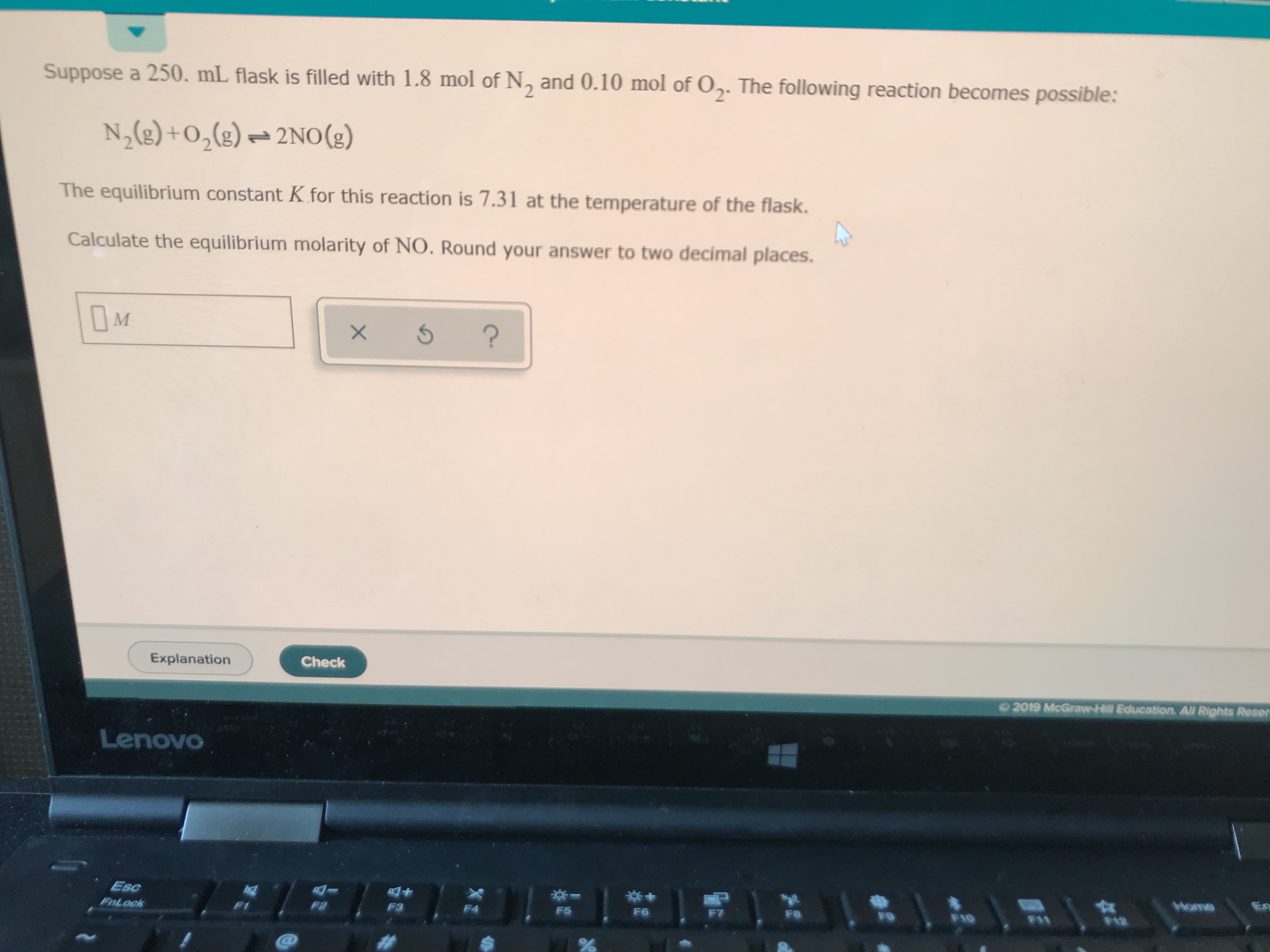# Suppose a 250 mL flask is filled with 1.8 mol N2 and 0.10 mol O2. The following reaction becomes possible: N2(g) + O2(g)⇆2NO(g) The equilibrium constant K for this reaction is 7.31 at the temperature of the flask. Calculate the equilibrium molarity of NO. Round your answer to two decimal places.